# Subtraction Facts to 20

Subtraction Facts to 20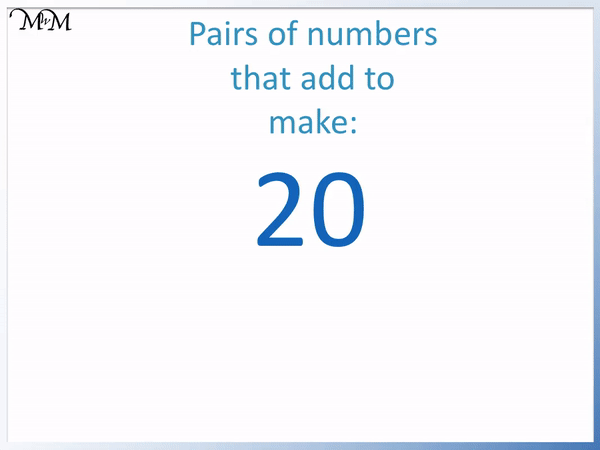• To subtract from 20 it helps to know the number bonds to 20.
• To learn the number bonds to 20, start with the number bonds to 10 and put a ‘1’ tens digit in front of one of the numbers in the pair.• 20 – 8 can be worked out using number bonds.
• The number 2 adds to 8 to make 10.
• 20 is ten larger than 10 and so, 12 adds to 8 to make 20.
• 10 – 8 = 2 and so, 20 – 8 = 12.

When subtracting from 20, the answer is ten more than if we had subtracted from 10.• Here we have 20 – something = 5.
• The missing number is the number that we add to 5 to make 20.
• 5 + 5 = 10 and so, 5 + 15 = 20.
• Therefore 20 – 15 = 5.
• The missing number is 15.
• The missing number is 10 larger than the number bond to 10 that goes with 5.# What are Subtraction Facts to 20?

Number facts to 20 are the pairs of numbers that add to make twenty and they are often written in the form of a sum.

Subtraction facts to 20 are subtraction sums in which we are subtracting from 20.

To subtract from 20 we should know the number bonds to 20. The number bonds to 20 are pairs of numbers that add to make 20.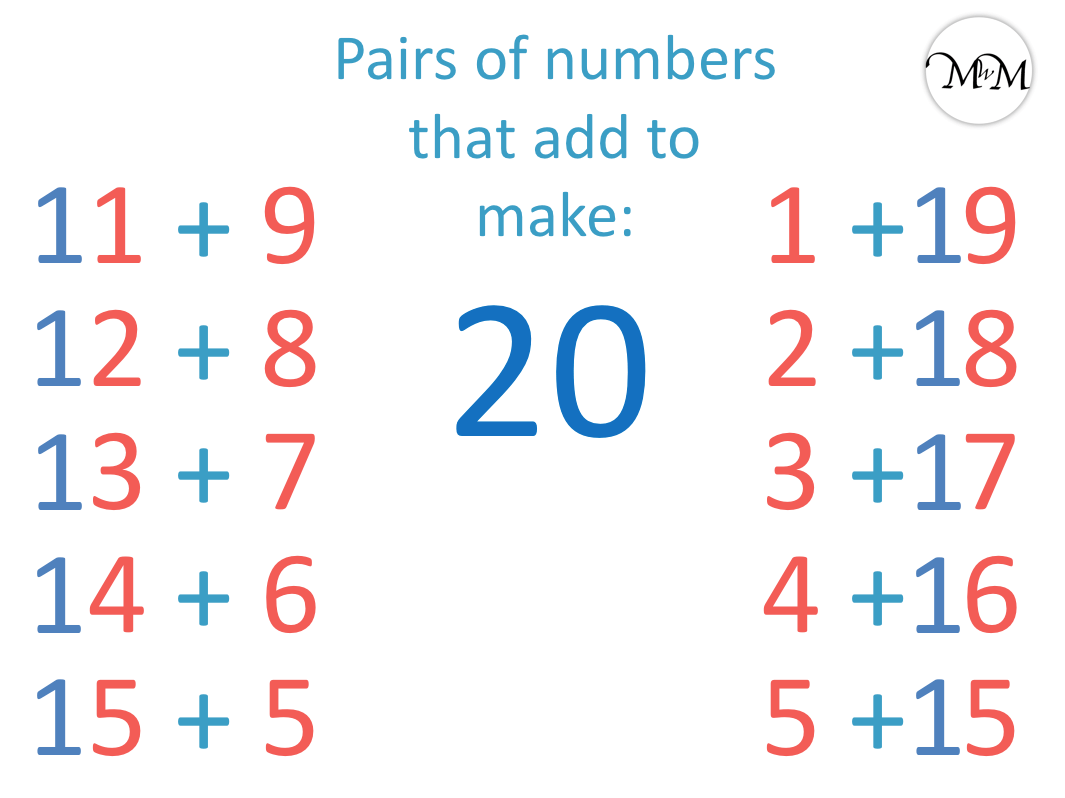To learn the number bonds to 20, start with the number bonds to 10 and add a ten to one of the numbers in each pair. We do this by adding a ‘1’ tens digit in front of one of the numbers.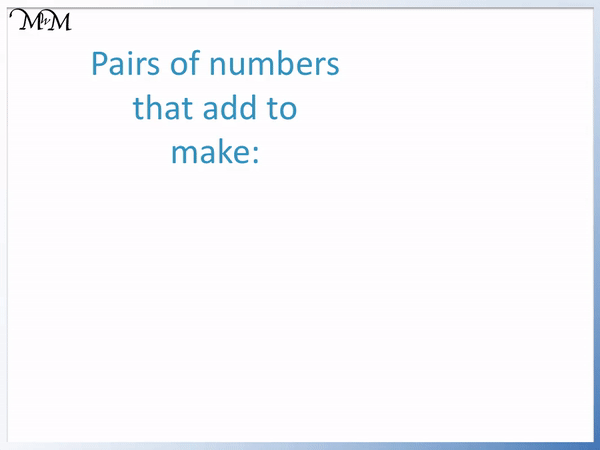Here is our first example of subtracting from 20.

We have 20 – 8.

From the number bonds to 10 we know that 8 + 2 = 10.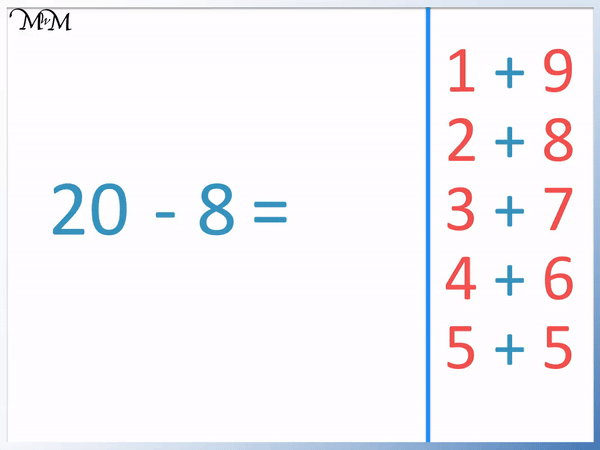8 + 2 = 10 and so, 8 + 12 = 20.

Because 8 + 12 = 20, 20 – 8 = 12.

The missing number in this subtraction fact is 12.

To subtract a number from 20, we can see how many more we add to this number to make 20. To subtract a single digit number from 20, the answer will be 10 more than this number’s number bond to 10.

For example, we subtracted 8 from 20 and so we add 10 to the number that adds to 8 to make 10. We add 10 to 2 to get the answer of 12.

Here is another example of subtracting from 20. We have 20 – 6.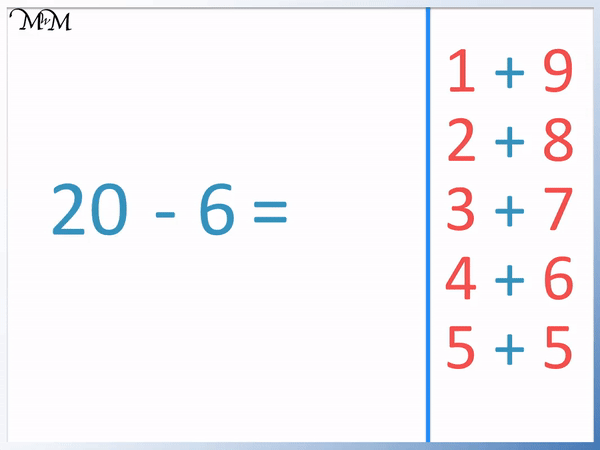Using the number bonds to 10, 6 + 4 = 10.

Therefore 6 + 14 = 20.

And so 20 – 6 = 14.

The missing number is 14, which is 10 more than 6’s number bond to ten.

In this example we will subtract a 2-digit number from 20. We have 20 – 12.We can see that 12 ends in a ‘2’.

2 + 8 = 10

And so, 12 + 8 = 20.

The answer to 20 – 12 is 8.

To subtract a 2-digit number from 20, the answer will be the number bond to ten of the number that is 10 less than the number being subtracted.

In this example we are subtracting 12 and so the answer is the number bond to ten that goes with 2.

In this example we are finding the missing number in the middle of a subtraction fact to 20.

20 – something = 5.

The missing number is the number that can be added to 5 to make 20.5 + 5 = 10 and so,

5 + 15 = 20.

The missing number is 10 more than the number bond to ten of the answer.

Here is an example of 20 – something = 3.We start by looking at the answer to the subtraction, which is 3.

3 + 7 = 10 and so,

3 + 17 = 20.

Therefore 20 – 17 = 3.

The missing number is 17, which is ten more than 3’s number bond to ten.Now try our lesson on Addition using the Compensation Strategy where we learn how to add numbers using the compensation strategy.